https://doi.org/10.5194/acp-20-3999-2020
https://doi.org/10.5194/acp-20-3999-2020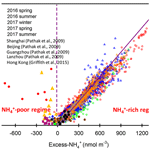# Heterogeneous formation of particulate nitrate under ammonium-rich regimes during the high-PM2.5 events in Nanjing, China

Yu-Chi Lin, Yan-Lin Zhang, Mei-Yi Fan, and Mengying Bao
Abstract

Particulate nitrate (${\mathrm{NO}}_{\mathrm{3}}^{-}$) not only influences regional climates but also contributes to the acidification of terrestrial and aquatic ecosystems. In 2016 and 2017, four intensive online measurements of water-soluble ions in PM2.5 were conducted in Nanjing City in order to investigate the potential formation mechanisms of particulate nitrate. During the sampling periods, ${\mathrm{NO}}_{\mathrm{3}}^{-}$ was the predominant species, accounting approximately for 35 % of the total water-soluble inorganic ions, followed by ${\mathrm{SO}}_{\mathrm{4}}^{\mathrm{2}-}$ (33 %) and ${\mathrm{NH}}_{\mathrm{4}}^{+}$ (24 %). Significant enhancements of nitrate aerosols in terms of both absolute concentrations and relative abundances suggested that ${\mathrm{NO}}_{\mathrm{3}}^{-}$ was a major contributing species to high-PM2.5 events (hourly PM2.5≥150µg m−3). High ${\mathrm{NO}}_{\mathrm{3}}^{-}$ concentrations mainly occurred under ${\mathrm{NH}}_{\mathrm{4}}^{+}$-rich conditions, implying that the formation of nitrate aerosols in Nanjing involved NH3. During the high-PM2.5 events, the nitrogen conversion ratios (Fn) were positively correlated with the aerosol liquid water content (ALWC; R>0.72 and p<0.05). Meanwhile, increasing ${\mathrm{NO}}_{\mathrm{3}}^{-}$ concentrations regularly coincided with increasing ALWC and decreasing Ox (${\mathrm{O}}_{x}={\mathrm{O}}_{\mathrm{3}}+{\mathrm{NO}}_{\mathrm{2}}$). These results suggested that the heterogeneous reaction was probably a major mechanism of nitrate formation during the high-PM2.5 events. Moreover, the average production rate of ${\mathrm{NO}}_{\mathrm{3}}^{-}$ by heterogeneous processes was estimated to be 12.6 % h−1 (4.1 µg m−3 h−1), which was much higher than that (2.5 % h−1; 0.8 µg m−3 h−1) of gas-phase reactions. This can also explain the abrupt increases in nitrate concentrations during the high-PM2.5 events. Utilizing the ISORROPIA II model, we found that nitrate aerosol formation in Nanjing during the high-PM2.5 events was HNO3 limited. This indicated that control of NOx emissions will be able to efficiently reduce airborne particulate nitrate concentrations and improve the air quality in this industrial city.

Share
Dates
1 Introduction

Due to the rapid growth of industrialization and urbanization, particulate matter (PM) pollution has become a severe problem in China in recent years (Chan and Yao, 2008; Zhang and Cao, 2015). Fine-mode particles (PM2.5, with aerodynamic diameters less than 2.5 µm) exhibit smaller sizes and contain many toxins emitted from anthropogenic emissions (Huang et al., 2018). PM2.5 easily penetrates the upper respiratory tract and is deposited into the human body, causing serious threats to human health. Numerous previous studies have proven that people exposed to high PM2.5 concentrations show increased risks of respiratory illness, cardiovascular diseases and asthma (Brauer et al., 2002; Defino et al., 2005), resulting in an increase in mortality (Nel, 2005).

Secondary inorganic aerosols (SIAs), including sulfate (${\mathrm{SO}}_{\mathrm{4}}^{\mathrm{2}-}$), nitrate (${\mathrm{NO}}_{\mathrm{3}}^{-}$) and ammonium (${\mathrm{NH}}_{\mathrm{4}}^{+}$), are major constituents of PM2.5, accounting for 25 %–60 % of the PM2.5 mass in urban cities of China (Huang et al., 2014; Wang et al., 2018; Yang et al., 2005; Ye et al., 2017; Zhao et al., 2013; Zou et al., 2018). Among these species, ${\mathrm{SO}}_{\mathrm{4}}^{\mathrm{2}-}$ and ${\mathrm{NO}}_{\mathrm{3}}^{-}$ are acidic ions, which tend to be neutralized by ${\mathrm{NH}}_{\mathrm{4}}^{+}$. Previously, many studies suggested that ${\mathrm{SO}}_{\mathrm{4}}^{\mathrm{2}-}$ dominated SIAs in urban cities of China (Kong et al., 2014; Tao et al., 2016; Yang et al., 2005; Yao et al., 2002; Zhao et al., 2013). In recent years, the Chinese government reduced its anthropogenic emissions by 62 % and 17 % for SO2 and NOx, respectively (Zheng et al., 2018). This revealed that the reduction efficiencies of SO2 emissions were much higher than those of NOx. Consequently, nitrate has become the dominant species of SIAs, especially during PM haze events (Wang et al., 2018; Wen et al., 2015; Zou et al., 2018).

In the atmosphere, ammonium nitrate (NH4NO3) is a major form of nitrate aerosols in fine-mode particles. NH4NO3 is a semivolatile species which partitions from the particle phase into the gas phase under high-temperature (T) conditions. It deliquesces when the ambient relative humidity (RH) is higher than its deliquescence relative humidity (DRH; nearly 62 % RH at atmospheric standard condition). To produce NH4NO3, nitrogen oxides (NOx) and ammonia (NH3) undergo a series of chemical reactions. NOx mostly emits as fresh NO, which is subsequently oxidized to NO2 and reacts with hydroxyl (OH) radicals to generate nitric acid (HNO3), and then HNO3 reacts with NH3 to yield NH4NO3 particles, as listed in Reactions (R1) and (R2) (Calvert and Stockwell, 1983). Particulate NH4NO3 formation rate is profoundly dependent on the ambient T and RH since both parameters influence the equilibrium constant of ${\mathrm{NO}}_{\mathrm{3}}^{-}$ and ${\mathrm{NH}}_{\mathrm{4}}^{+}$ between the particle and gas phases, as listed in Reaction (R2) (Lin and Cheng, 2007).

$\begin{array}{}\text{(R1)}& & {\mathrm{NO}}_{\mathrm{2}\left(\mathrm{g}\right)}+{\mathrm{OH}}_{\left(\mathrm{g}\right)}\to {\mathrm{HNO}}_{\mathrm{3}\left(\mathrm{g}\right)}\phantom{\rule{0.25em}{0ex}}{k}_{\mathrm{1}}\text{(R2)}& & {\mathrm{HNO}}_{\mathrm{3}\left(\mathrm{g}\right)}+{\mathrm{NH}}_{\mathrm{3}\left(\mathrm{g}\right)}\to {\mathrm{NH}}_{\mathrm{4}}{\mathrm{NO}}_{\mathrm{3}\left(\mathrm{s},\phantom{\rule{0.125em}{0ex}}\mathrm{aq}\right)}\phantom{\rule{0.25em}{0ex}}{k}_{\mathrm{2}}\end{array}$

Here, k1 and k2 are the reaction rate and equilibrium constant of Reactions (R1) and (R2), respectively. The equilibrium constant k2 can be expressed as the product of HNO3 and NH3.

Heterogeneous reactions have been considered an important mechanism of nitrate formation during nighttime. As listed in Reaction (R3), liquid HNO3 is produced by the hydrolysis of dinitrogen pentoxide (N2O5) on aerosol surfaces (Brown and Stutz, 2012; Chang et al., 2011; Mental et al., 1999; Wahner et al., 1998). Liquid HNO3 can be neutralized by ${\mathrm{NH}}_{\mathrm{4}}^{+}$, which is produced from the conversion of gaseous NH3. Nitrate aerosols yielded from both Reactions (R2) and (R3) require NH3, and therefore these processes of ${\mathrm{NO}}_{\mathrm{3}}^{-}$ formation occur under NH4-rich conditions. Sometimes, there is not enough NH3 (${\mathrm{NH}}_{\mathrm{4}}^{+}$) to react with (to be neutralized by) HNO3 (${\mathrm{NO}}_{\mathrm{3}}^{-}$) after complete neutralization by H2SO4. Under this condition, HNO3 tends to react with (or to be neutralized by) other alkaline species such as Ca-rich dust (CaCO3), and subsequently, nitrate aerosol is produced under a ${\mathrm{NH}}_{\mathrm{4}}^{+}$-poor regime (Goodman et al., 2000).

$\begin{array}{}\text{(R3)}& {\mathrm{N}}_{\mathrm{2}}{\mathrm{O}}_{\mathrm{5}\left(\mathrm{g}\right)}+{\mathrm{H}}_{\mathrm{2}}{\mathrm{O}}_{\left(\mathrm{l}\right)}\to \mathrm{2}{\mathrm{HNO}}_{\mathrm{3}\left(\mathrm{aq}\right)}\end{array}$

The Yangtze River Delta (YRD) region is one of the well-known polluted areas in China (Zhang and Cao, 2015). Different from the case of dramatic elevated sulfate aerosol levels in Beijing (Wang et al., 2016), nitrate aerosols seem to be a major contributing species during haze days in the YRD region (Wang et al., 2015, 2018). The formation mechanisms of nitrate in Nanjing have not yet been well understood, especially during high-PM events. In this study, four intensive online measurements of water-soluble ions in PM2.5 were conducted in Nanjing City in 2016 and 2017. The data provided information on the hourly evolution of water-soluble inorganic ions (WSIIs) in the industrial city. The ${\mathrm{NO}}_{\mathrm{3}}^{-}$ distributions under different ${\mathrm{NH}}_{\mathrm{4}}^{+}$ regimes (${\mathrm{NH}}_{\mathrm{4}}^{+}$-poor and ${\mathrm{NH}}_{\mathrm{4}}^{+}$-rich conditions) were also discussed. Finally, we investigated the potential formation mechanisms of nitrate aerosols and their production rates during high-PM2.5 events based on the online measurements.

2 Methodology

## 2.1 Sampling site

Particulate WSIIs and inorganic gases were continuously monitored at Nanjing University of Information Science and Technology (NUIST), located in the northern part of Nanjing City (see Fig. S1 in the Supplement). In addition to vehicle emissions, petroleum chemical refineries and steel manufacturing plants situated in the northeast and east direction at a distance of approximately 5 km are also major anthropogenic emissions near the receptor site. Four intensive campaigns were conducted from March 2016 to August 2017. During each experiment, the hourly concentrations of WSIIs in PM2.5 and inorganic gases were continuously observed. Meanwhile, the hourly PM2.5 mass, NO2 and O3 concentrations, and the ambient T and RH were acquired from the Pukou air quality monitoring station, which is located to the southwest of the receptor site.

## 2.2 Instruments

To monitor the hourly concentrations of WSIIs (Cl, ${\mathrm{NO}}_{\mathrm{3}}^{-}$, ${\mathrm{SO}}_{\mathrm{4}}^{\mathrm{2}-}$, Na+, ${\mathrm{NH}}_{\mathrm{4}}^{+}$, K+, Mg2+ and Ca2+), an online Monitor for AeRosols and Gases in ambient Air (MARGA; Applikon-ENC, the Netherlands) instrument with a PM2.5 inlet was employed. Using this instrument, the WSIIs in PM2.5 were collected by a stream jet aerosol collector, while acidic (HCl, HONO, HNO3 and SO2) and basic gases (NH3) were dissolved in a hydrogen peroxide solution on a wet rotation denuder (ten Brink et al., 2007; Griffith, et al., 2015). The liquid samples were then collected with syringe pumps and analyzed by ion chromatography (IC). Before each campaign, a seven-point calibration curve of each species was made, and an internal standard solution (LiBr) was used to check instrumental drifts. The method detection limits (MDLs) of Cl, ${\mathrm{NO}}_{\mathrm{3}}^{-}$, ${\mathrm{SO}}_{\mathrm{4}}^{\mathrm{2}-}$, Na+, ${\mathrm{NH}}_{\mathrm{4}}^{+}$, K+, Mg2+ and Ca2+ were, 0.01, 0.04, 0.06, 0.05, 0.05, 0.07, 0.05 and 0.11 µg m−3, respectively. For gases, the MDLs were 0.07, 0.09, 0.06, 0.02 and 0.08 µg m−3 for HCl, HONO, HNO3, SO2 and NH3, respectively.

## 2.3 ISORROPIA II model

In this work, we used the ISORROPIA II model to calculate the aerosol liquid water content (ALWC). ISORROPIA II is a thermodynamic equilibrium model which is built based on the Na+ClCa2+K+Mg2+${\mathrm{SO}}_{\mathrm{4}}^{\mathrm{2}-}$${\mathrm{NH}}_{\mathrm{4}}^{+}$${\mathrm{NO}}_{\mathrm{3}}^{-}$H2O aerosol system (Fountoukis and Nenes, 2007). This model has been successfully used to estimate the liquid water content in aerosols with uncertainty of ∼20 % compared to the observed ALWC (Bian et al., 2014; Guo et al., 2015; Liu et al., 2017). This underestimation might be due to the missed species in ISORROPIA II, organic aerosols, which contributed approximately 35 % to total ALWC (Guo et al., 2015). Here, the model was computed as a “forward problem”, in which the quantities of aerosol- and gas-phase compositions along with the T and RH were well known. Additionally, the modeled values were determined using the “metastable” mode, which indicated that the aerosol compositions were assumed to be composed of an aqueous solution (Liu et al., 2017). The details of this model can be found elsewhere (Fountoukis and Nenes, 2007). In this work, the observed concentrations of total nitrate (${\mathrm{HNO}}_{\mathrm{3}}+{\mathrm{NO}}_{\mathrm{3}}^{-}$), total ammonium (${\mathrm{NH}}_{\mathrm{3}}+{\mathrm{NH}}_{\mathrm{4}}^{+}$), total chloride ($\mathrm{HCl}+{\mathrm{Cl}}^{-}$), ${\mathrm{SO}}_{\mathrm{4}}^{\mathrm{2}-}$, Na+, K+, Mg2+ and Ca2+ along with measured ambient T and RH served as input of ISORROPIA II model.

## 2.4 Potential source contribution function

Potential source contribution function (PSCF) is a method to identify the potential source regions of air pollutants. It has also been widely used to differentiate local emission from long-range transported sources (Zhang et al., 2013; Hui et al., 2018) based on the trajectory analysis calculated from GDAS (Global Data Assimilation System), which processed by the National Centers for Environmental Prediction (NCEP). The zone of concern is divided into i×j small equal grid cells, and then PSCF in the (i,j)th cell (PSCFij) can be defined as (Polissar et al., 1999)

$\begin{array}{}\text{(1)}& {\mathrm{PSCF}}_{ij}=\frac{{m}_{ij}}{{n}_{ij}},\end{array}$

where mij is the number of “high-nitrate pollution” trajectory endpoints in the (i,j)th cell and nij is the total number of trajectory endpoints fallen into the (i,j)th cell. In this study, the 80th-percentile value of nitrate concentration was treated as the high-nitrate pollution threshold. To reduce the uncertainty caused by the small values of nij, the weighting function of Wij has to be considered (Polissar et al., 1999).

$\begin{array}{}\text{(2)}& {W}_{ij}=\left[\begin{array}{l}\mathrm{1.00}\phantom{\rule{0.25em}{0ex}};\phantom{\rule{0.25em}{0ex}}\mathrm{80}<{n}_{ij}\\ \mathrm{0.70}\phantom{\rule{0.25em}{0ex}};\phantom{\rule{0.25em}{0ex}}\mathrm{20}<{n}_{ij}\le \mathrm{80}\\ \mathrm{0.42}\phantom{\rule{0.25em}{0ex}};\phantom{\rule{0.25em}{0ex}}\mathrm{10}<{n}_{ij}\le \mathrm{20}\\ \mathrm{0.05}\phantom{\rule{0.25em}{0ex}};\phantom{\rule{0.25em}{0ex}}{n}_{ij}\le \mathrm{10}\end{array}\right]\end{array}$

In this study, the domain of the study area was in a range of 20–55 N, 105–135 E; the resolution of grid cell was 0.5× 0.5.

3 Results and discussion

## 3.1 Overview of water-soluble inorganic ions

Four intensive online measurements of WSIIs in PM2.5 were carried out in Nanjing City from March 2016 to August 2017. Figure 1a plots the time series of the hourly PM2.5 mass concentrations during the sampling periods. As seen, the hourly PM2.5 mass concentrations varied from 5 to 252 µg m−3 with a mean value of 58±35µg m−3. Compared with the 24 h guideline (25 µg m−3) suggested by the World Health Organization (WHO), our average PM2.5 concentration (58 µg m−3) was 2.3 times higher. This indicated that PM pollution in Nanjing City was a serious problem. During the campaigns, several high-PM2.5 events with hourly PM2.5 concentrations of higher than 150 µg m−3 were observed in the springtime and wintertime. These high PM2.5 levels lasted for more than 3 h, with obviously elevated ${\mathrm{NO}}_{\mathrm{3}}^{-}$. The details of nitrate formation during the high-PM2.5 hours will be discussed in the following sections.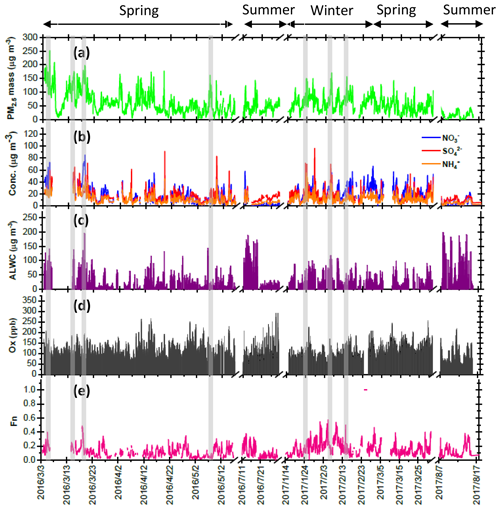Figure 1Time series of concentrations in (a) PM2.5 mass, (b) SIA species, (c) ALWC and (d) Ox along with (e) Fn observed in Nanjing during the sampling periods. The grey shadows represent the high-PM2.5 periods discussed in the Sect. 3.6.

Figure 1b shows time series of the hourly concentrations of SIA species, including ${\mathrm{SO}}_{\mathrm{4}}^{\mathrm{2}-}$, ${\mathrm{NO}}_{\mathrm{3}}^{-}$ and ${\mathrm{NH}}_{\mathrm{4}}^{+}$. The lack of data from 7 to 14 March 2016 was due to a malfunction of the MARGA instrument. During the sampling periods, the ${\mathrm{NO}}_{\mathrm{3}}^{-}$ concentrations varied from 0.1 to 85.1 µg m−3 with a mean value of 16.7±12.8µg m−3. The ${\mathrm{SO}}_{\mathrm{4}}^{\mathrm{2}-}$ concentrations ranged from 1.7 to 96.2 µg m−3 and averaged 14.9±9.1µg m−3. The ${\mathrm{NH}}_{\mathrm{4}}^{+}$ concentrations fluctuated between 0.8 and 44.9 µg m−3 with a mean value of 10.7±6.7µg m−3. On average, SIAs accounted for 91.2 % of the total water-soluble inorganic ions (TWSIIs) during the entirety of the sampling periods (see Fig. 2a). Among these species, ${\mathrm{NO}}_{\mathrm{3}}^{-}$ accounted for 34.7 % of the TWSIIs, followed by ${\mathrm{SO}}_{\mathrm{4}}^{\mathrm{2}-}$ (32.6 %) and ${\mathrm{NH}}_{\mathrm{4}}^{+}$ (23.9 %). The abundances of other ions, including Cl, K+, Ca2+, Na+ and Mg2+, were 4.9 %, 1.8 %, 1.3 %, 0.7 % and 0.3 %, respectively. Figure S2 shows the scatter plot of the equivalent concentrations of the cations (Na+, ${\mathrm{NH}}_{\mathrm{4}}^{+}$, K+, Mg2+ and Ca2+) and anions (Cl, ${\mathrm{SO}}_{\mathrm{4}}^{\mathrm{2}-}$ and ${\mathrm{NH}}_{\mathrm{4}}^{+}$). As seen, good correlations (R= 0.98–0.99, with a significance level p<0.05) between cations and anions were found during the various sampling periods. The ratio of cation to anion was very close to 1.0 during each season, reflecting an ionic balance. This also indicated that our data exhibited good quality and was able to be used for the further analysis of scientific issues.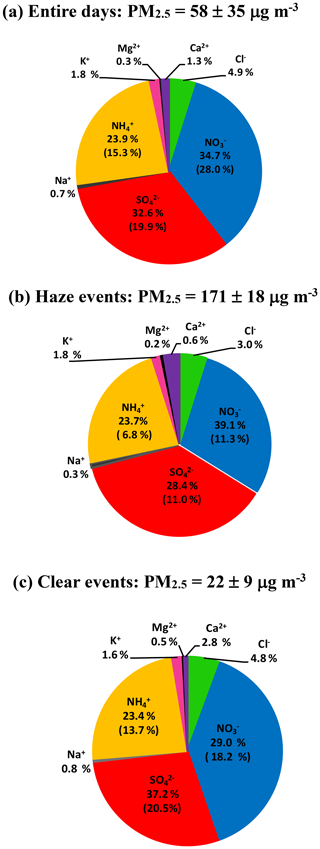Figure 2Abundance of each species in TWSIIs during the (a) entire, (b) haze (PM2.5 150 µg m−3) and (c) clear (PM2.5< 35 µg m−3) events. The numbers in the parentheses are standard deviations.

All SIA species exhibited similar seasonal patterns, with lower concentrations in the summer, especially for ${\mathrm{NO}}_{\mathrm{3}}^{-}$. The average concentrations of nitrate were 6.7 and 5.7 µg m−3 in the summertime of 2016 and 2017, respectively (see Fig. S3). These values were much lower than those observed during other seasons. The local meteorological conditions, which were favorable for the dilution of air pollution, were one of the reasons for the declined ${\mathrm{NO}}_{\mathrm{3}}^{-}$ concentrations during the hot seasons (Zhang and Cao, 2015). Another important reason for this effect was attributed to the formation process of PM2.5 nitrate, which is very sensitive to the ambient T and RH (Lin and Cheng, 2007). Figure S4a depicts the theoretical equilibrium constants of partitioned ${\mathrm{NO}}_{\mathrm{3}}^{-}$ and ${\mathrm{NH}}_{\mathrm{4}}^{+}$ between the particle and gas phase (${\mathrm{HNO}}_{\mathrm{3}\left(\mathrm{g}\right)}+{\mathrm{NH}}_{\mathrm{3}\left(\mathrm{g}\right)}\to {\mathrm{NH}}_{\mathrm{4}}{\mathrm{NO}}_{\mathrm{3}\left(\mathrm{s},\phantom{\rule{0.125em}{0ex}}\mathrm{aq}\right)}$ as seen in Reaction R2) under different T and RH conditions. The details of calculation approach of the theoretical equilibrium constants are described in the Supplement, Sect. S1. Note that the y axis is presented on a log scale. The theoretical equilibrium constants increased exponentially with increasing ambient temperature but decreased with increasing RH. This indicated that NH4NO3 would be partitioned into the gas phase due to high equilibrium constants under high-temperature and low-RH conditions. Figure S4b illustrates the time series of the theoretical and observed equilibrium constants during the sampling periods. As can be seen, most of the observed equilibrium constants were higher than the theoretical ones, suggesting that NH4NO3 aerosols were produced in Nanjing during the sampling periods. Obviously, higher theoretical and lower observed equilibrium constants were found during the summer. This suggested that more ${\mathrm{NO}}_{\mathrm{3}}^{-}$ and ${\mathrm{NH}}_{\mathrm{4}}^{+}$ would tend to be partitioned into the gas phase, resulting in lower particulate nitrate concentrations during hot seasons (Lin and Cheng, 2007).

Apart from seasonal variations, pronounced diurnal patterns were also found for SIA species (see Fig. 3). ${\mathrm{NO}}_{\mathrm{3}}^{-}$ exhibited similar diel cycles during different seasons, with higher concentrations in the early morning (03:00–08:00 LT) and lower levels between 14:00 and 17:00 LT. The high nitrate concentrations in the early morning might be caused by the nitrate formation via heterogeneous reaction in the dark and gas-phase oxidation after sunrise and the subsequent condensation on pre-existing particles before the temperature increased and RH decreased afterwards. Moreover, the lower planet boundary layer (PBL) might be another reason for enhanced nitrate in the early morning. However, the lower concentrations of nitrate during the daytime might be attributed to the higher PBL and high temperatures, which inhibited the build-up of nitrate, especially during the summertime. In terms of sulfate, higher concentrations were observed between 06:00 and 13:00 LT, indicating that the formation rate of sulfate was higher than the removal/dilution rate, leading to an increase in the sulfate concentration during the daytime. The diurnal patterns of ${\mathrm{NH}}_{\mathrm{4}}^{+}$ mimicked those of ${\mathrm{NO}}_{\mathrm{3}}^{-}$, showing lower concentrations during the daytime. This was explained by the drastic decrease in particulate NH4NO3 concentrations under high temperatures and low relative humidity, resulting in lower ${\mathrm{NH}}_{\mathrm{4}}^{+}$ levels during the daytime.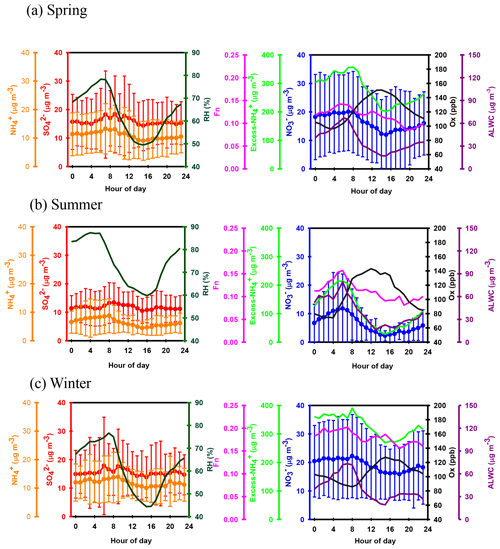Figure 3Diurnal variations in the concentrations of ${\mathrm{NO}}_{\mathrm{3}}^{-}$, ${\mathrm{SO}}_{\mathrm{4}}^{\mathrm{2}-}$ and ${\mathrm{NH}}_{\mathrm{4}}^{+}$, excess ${\mathrm{NH}}_{\mathrm{4}}^{+}$, Ox and ALWC, and nitrogen conversion ratio (Fn) and ambient relative humidity in Nanjing during the sampling periods. For ${\mathrm{SO}}_{\mathrm{4}}^{\mathrm{2}-}$, ${\mathrm{NO}}_{\mathrm{3}}^{-}$ and ${\mathrm{NH}}_{\mathrm{4}}^{+}$, the mean values (dots) and standard deviations (solid lines) are plotted.

## 3.2 Enhancements of nitrate at high PM2.5 levels

Figure S5 shows the scatter plots of ${\mathrm{NO}}_{\mathrm{3}}^{-}$, ${\mathrm{SO}}_{\mathrm{4}}^{\mathrm{2}-}$ and ${\mathrm{NH}}_{\mathrm{4}}^{+}$ against PM2.5. As seen, the slopes of ${\mathrm{NO}}_{\mathrm{3}}^{-}$ (${\mathrm{NO}}_{\mathrm{3}}^{-}$ vs. PM2.5 mass), ${\mathrm{SO}}_{\mathrm{4}}^{\mathrm{2}-}$ and ${\mathrm{NH}}_{\mathrm{4}}^{+}$ were 0.30, 0.24 and 0.19, respectively. This suggested that the increasing rate of ${\mathrm{NO}}_{\mathrm{3}}^{-}$ during the high-PM2.5 events was higher than those of other SIA species. At high PM2.5 levels (PM2.5≥150µg m3), ${\mathrm{NO}}_{\mathrm{3}}^{-}$, ${\mathrm{SO}}_{\mathrm{4}}^{\mathrm{2}-}$ and ${\mathrm{NH}}_{\mathrm{4}}^{+}$ contributed 39.1 %, 28.4 % and 23.7 % of the TWSIIs, respectively (Fig. 2b). However, the relative abundances of ${\mathrm{NO}}_{\mathrm{3}}^{-}$, ${\mathrm{SO}}_{\mathrm{4}}^{\mathrm{2}-}$ and ${\mathrm{NH}}_{\mathrm{4}}^{+}$ during low PM2.5 concentrations (hourly PM2.5 <35µg m−3; see Fig. 2c) were 29.0 %, 37.2 % and 23.4 %, respectively. In recent years, dramatically enhanced amounts of nitrate aerosols during high-PM events have been observed at many urban sites in China (Wen et al., 2015; Wang et al., 2017, 2018; Zou et al., 2018). For instance, Zou et al. (2018) found that the nitrate concentrations during the occurrence of polluted air in Beijing and Tianjin were almost 14 times higher than those on relatively clean days (PM2.5<75µg m−3), and the enhancement ratio of nitrate was much higher than that (5.3) of sulfate. Wang et al. (2018) noted that the enhancement ratio of ${\mathrm{NO}}_{\mathrm{3}}^{-}$ (∼6) between haze and clear days in Ningbo of the YRD region was much higher than that of ${\mathrm{SO}}_{\mathrm{4}}^{\mathrm{2}-}$ (∼3). These findings suggested that ${\mathrm{NO}}_{\mathrm{3}}^{-}$ was a major contributing species to fine particles during haze days since its increasing ratio between haze and non-haze days was much higher than those of other SIA species, such as sulfate and ammonium.

## 3.3 PSCF result of high-nitrate pollution

During the high-PM2.5 pollution, significant enhanced nitrate aerosols in terms of both absolute concentration and relative abundance to TWSIIs were found. Next, we tried to use PSCF analysis to identify whether local emission or long-range transported air pollution was the major source of high nitrate concentrations at the receptor site. In this work, the 80th-percentile values of nitrate concentration was selected as high-nitrate pollution threshold for PSCF analysis. Figure 4 plots the PSCF result of the high-nitrate pollution in Nanjing during the sampling periods. The region corresponding to the high-PSCF-value grid is a potential source region of nitrate aerosols. As can be seen, the areas with a high PSCF value (>0.8) were regularly local areas surrounding by Nanjing, while PSCF values from other long-distance areas were lower than 0.2. This suggested that ${\mathrm{NO}}_{\mathrm{3}}^{-}$ aerosols in Nanjing during the high-nitrate pollution were likely from local emissions rather than long-range transported sources.

## 3.4 Nitrate formation under different ammonium regimes

Ammonium is a major species that neutralizes particulate ${\mathrm{SO}}_{\mathrm{4}}^{\mathrm{2}-}$ and ${\mathrm{NO}}_{\mathrm{3}}^{-}$. In the atmosphere, ${\mathrm{SO}}_{\mathrm{4}}^{\mathrm{2}-}$ competes with ${\mathrm{NO}}_{\mathrm{3}}^{-}$ for ${\mathrm{NH}}_{\mathrm{4}}^{+}$ during their formation processes, and therefore, the relationship between the molar ratios of ${\mathrm{NO}}_{\mathrm{3}}^{-}/{\mathrm{SO}}_{\mathrm{4}}^{\mathrm{2}-}$ and ${\mathrm{NH}}_{\mathrm{4}}^{+}/{\mathrm{SO}}_{\mathrm{4}}^{\mathrm{2}-}$ can give us a hint for understanding the formation of ${\mathrm{NO}}_{\mathrm{3}}^{-}$ under different ammonium regimes (Pathak et al., 2009; He et al., 2012; Tao et al., 2016). In an ammonium-rich regime, the HNO3 produced by both gas oxidation and heterogeneous process reacts with (or neutralizes) “excess-ammonium” (excess-${\mathrm{NH}}_{\mathrm{4}}^{+}$) cation at a ${\mathrm{NH}}_{\mathrm{4}}^{+}/{\mathrm{SO}}_{\mathrm{4}}^{\mathrm{2}-}$ molar ratio >2 (theoretical value in an ${\mathrm{NH}}_{\mathrm{4}}^{+}$-rich regime) when sulfate is completely neutralized by ${\mathrm{NH}}_{\mathrm{4}}^{+}$ to form (NH4)2SO4 (Squizzato et al., 2013; Ye et al., 2011). In contrast, nitrate can be found under ammonium-poor conditions with a theoretical ${\mathrm{NH}}_{\mathrm{4}}^{+}/{\mathrm{SO}}_{\mathrm{4}}^{\mathrm{2}-}$ value that should be less than 2 (Pathak et al., 2009). Under ${\mathrm{NH}}_{\mathrm{4}}^{+}$-poor conditions, HNO3 reacts with other cations, such as calcium carbonate, frequently found in natural dust.

Figure 5 shows the scatter plot of the molar ratios of ${\mathrm{NO}}_{\mathrm{3}}^{-}/{\mathrm{SO}}_{\mathrm{4}}^{\mathrm{2}-}$ against ${\mathrm{NH}}_{\mathrm{4}}^{+}/{\mathrm{SO}}_{\mathrm{4}}^{\mathrm{2}-}$. It is found that good correlations existed between ${\mathrm{NO}}_{\mathrm{3}}^{-}/{\mathrm{SO}}_{\mathrm{4}}^{\mathrm{2}-}$ and ${\mathrm{NH}}_{\mathrm{4}}^{+}/{\mathrm{SO}}_{\mathrm{4}}^{\mathrm{2}-}$ under ${\mathrm{NH}}_{\mathrm{4}}^{+}$-rich regimes, with a coefficient of determination (R2) of 0.84–0.94 in the different seasons (see in Table 1). Utilizing the linear regression model, we suggested that nitrate aerosols (in ${\mathrm{NH}}_{\mathrm{4}}^{+}$-rich regimes) began to form when the ${\mathrm{NH}}_{\mathrm{4}}^{+}/{\mathrm{SO}}_{\mathrm{4}}^{\mathrm{2}-}$ molar ratios exceeded the criterion values of 1.7–2.0 during the different seasons (Table 1). The criterion value can be calculated as absolute value of “intercept” dividing by slope in each linear regression model (He et al., 2012). The criterion values below 2 suggested that part of the sulfate might have existed in other forms, such as ammonium bisulfate. On the other hand, under ammonium-rich conditions, nitrate concentrations should be positively proportional to “excess-${\mathrm{NH}}_{\mathrm{4}}^{+}$” concentrations, a relationship which was defined as [excess-${\mathrm{NH}}_{\mathrm{4}}^{+}$] = (${\mathrm{NH}}_{\mathrm{4}}^{+}/{\mathrm{SO}}_{\mathrm{4}}^{\mathrm{2}-}$ criterion value) × [${\mathrm{SO}}_{\mathrm{4}-}^{\mathrm{2}-}$] (Pathak et al., 2009) (sulfate is in units of nanomoles per cubic meter here). The criterion values were acquired from the regression models, as listed in Table 1. The results revealed that the excess-${\mathrm{NH}}_{\mathrm{4}}^{+}$ concentrations varied from −283 to 1422 nmol m−3 (see Fig. 6), and only 1 % of data showed deficit-${\mathrm{NH}}_{\mathrm{4}}^{+}$ conditions, reflecting that ${\mathrm{NO}}_{\mathrm{3}}^{-}$ formation in Nanjing occurred primarily under the ${\mathrm{NH}}_{\mathrm{4}}^{+}$-rich conditions. Moreover, the excess ${\mathrm{NH}}_{\mathrm{4}}^{+}$ had apparent diurnal cycles, with higher concentrations in the early morning and lower concentrations at midday and in the early afternoon (see Fig. 3, where we converted the units from nanomoles per cubic meter to micrograms per cubic meter). The diurnal patterns of ${\mathrm{NO}}_{\mathrm{3}}^{-}$ mimicked those of the excess ${\mathrm{NH}}_{\mathrm{4}}^{+}$. This also suggested that particulate ${\mathrm{NO}}_{\mathrm{3}}^{-}$ formation occurred mainly under ${\mathrm{NH}}_{\mathrm{4}}^{+}$-rich conditions. Figure 6 illustrates the relationship between the nitrate and excess-${\mathrm{NH}}_{\mathrm{4}}^{+}$ molar concentrations during the sampling periods. The nitrate molar concentrations correlated linearly with the excess-${\mathrm{NH}}_{\mathrm{4}}^{+}$ molar concentrations with a slope of approximately 1.0, which was consistent with the molar ratio of reaction between HNO3 and NH3. Interestingly, some scattered points were found in high ammonium concentrations (excess ${\mathrm{NH}}_{\mathrm{4}}^{+}\ge \mathrm{900}$ nmol m−3 ∼16.2µg m−3), implying that residual ${\mathrm{NH}}_{\mathrm{4}}^{+}$ might be presented in another form such as NH4Cl under high-${\mathrm{NH}}_{\mathrm{4}}^{+}$ conditions. On the contrary, ${\mathrm{NO}}_{\mathrm{3}}^{-}$ aerosols can be produced without involving NH3; therefore, ${\mathrm{NO}}_{\mathrm{3}}^{-}$ did not correlate well with the excess ${\mathrm{NH}}_{\mathrm{4}}^{+}$ under a ${\mathrm{NH}}_{\mathrm{4}}^{+}$-poor regime.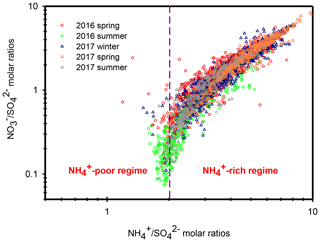Figure 5Scatter plots of molar ratios of ${\mathrm{NO}}_{\mathrm{3}}^{-}/{\mathrm{SO}}_{\mathrm{4}}^{\mathrm{2}-}$ against ${\mathrm{NH}}_{\mathrm{4}}^{+}/{\mathrm{SO}}_{\mathrm{4}}^{\mathrm{2}-}$ in Nanjing during the different seasons.

Table 1The regression models between ${\mathrm{NO}}_{\mathrm{3}}^{-}/{\mathrm{SO}}_{\mathrm{4}}^{\mathrm{2}-}$ (Y) and ${\mathrm{NH}}_{\mathrm{4}}^{+}/{\mathrm{SO}}_{\mathrm{4}}^{\mathrm{2}-}$ (X) along with the criterion values of ${\mathrm{NH}}_{\mathrm{4}}^{+}/{\mathrm{SO}}_{\mathrm{4}}^{\mathrm{2}-}$ in ammonium-rich regime during the sampling periods.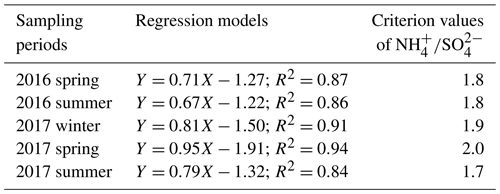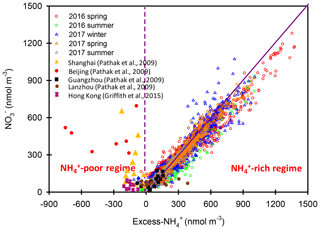Figure 6Scatter plots of ${\mathrm{NO}}_{\mathrm{3}}^{-}$ vs. excess-${\mathrm{NH}}_{\mathrm{4}}^{+}$ molar concentrations in Nanjing during the different seasons. The results in Beijing, Shanghai, Guangzhou, Lanzhou and Hong Kong are also shown in this figure.

In this study, high nitrate concentrations were always found under ${\mathrm{NH}}_{\mathrm{4}}^{+}$-rich regimes, elucidating that nitrate production during high PM levels in Nanjing had to be involved with NH3 or ${\mathrm{NH}}_{\mathrm{4}}^{+}$. Figure 6 also shows the nitrate concentrations against the excess-${\mathrm{NH}}_{\mathrm{4}}^{+}$ concentrations observed in various cities of China during the summertime (Pathak et al., 2009; Griffith et al., 2015). In Beijing and Shanghai, high nitrate concentrations during the summertime were found under ${\mathrm{NH}}_{\mathrm{4}}^{+}$-deficient conditions, which was very different from the findings of this work. In these studies (Pathak et al., 2009; Griffith et al., 2015), the high nitrate concentrations associated with ${\mathrm{NH}}_{\mathrm{4}}^{+}$-poor conditions might be due to the lower excess-${\mathrm{NH}}_{\mathrm{4}}^{+}$ concentrations under high-${\mathrm{SO}}_{\mathrm{4}}^{\mathrm{2}-}$ conditions at that time since the strict control of SO2 emissions by the Chinese government started in 2010 (Zheng et al., 2018). In recent years, the reduction of anthropogenic SO2 emissions decreased the airborne ${\mathrm{SO}}_{\mathrm{4}}^{\mathrm{2}-}$ concentrations, resulting in more excess-${\mathrm{NH}}_{\mathrm{4}}^{+}$ concentrations and leading to nitrate aerosol formation under ${\mathrm{NH}}_{\mathrm{4}}^{+}$-rich regimes. This argument can be supported by the recent results shown in Fig. S6, in which high nitrate concentrations in Beijing were always found under ${\mathrm{NH}}_{\mathrm{4}}^{+}$-rich regimes.

## 3.5 Nitrate formation mechanism during high-PM2.5 episodes

In this section, we attempt to explore the formation mechanisms of nitrate aerosols during high PM2.5 levels. Here, nitrogen conversion ratio (Fn) was used to evaluate the conversion capability of NO2 to total nitrate (TN; $\mathrm{TN}={\mathrm{HNO}}_{\mathrm{3}}+{\mathrm{NO}}_{\mathrm{3}}^{-}$), and it can be defined as (Khoder, 2002; Lin et al., 2006)

$\begin{array}{}\text{(3)}& {F}_{\mathrm{n}}=\frac{{\mathrm{GNO}}_{\mathrm{3}}^{-}+{\mathrm{PNO}}_{\mathrm{3}}^{-}}{{\mathrm{GNO}}_{\mathrm{3}}^{-}+{\mathrm{PNO}}_{\mathrm{3}}+{\mathrm{NO}}_{\mathrm{2}}},\end{array}$

where ${\mathrm{GNO}}_{\mathrm{3}}^{-}$ and ${\mathrm{PNO}}_{\mathrm{3}}^{-}$ represent the NO2 concentrations in nitric acid and particulate nitrate, respectively, with the units of micrograms per cubic meter. The results showed that the Fn values during the sampling periods varied from 0.01 to 0.57 with a mean value of 0.14±0.09 (see Fig. 1e). This value was comparable to that (0.17) in Taichung, Taiwan, where both gas-oxidation and heterogeneous reaction were the dominant formation mechanisms of atmospheric HNO3 (or ${\mathrm{NO}}_{\mathrm{3}}^{-}$) (Lin et al., 2006). However, our Fn value was 2.3 times higher than that (0.06) in Dokki, Egypt (Khoder, 2002). The reason of significant discrepancy of Fn between this work and that in Dokki was not clearly understood, but it might be attributed to different formation processes of HNO3. In Dokki, gas-phase oxidation was the dominant pathway of HNO3 production, while the heterogeneous process (Reaction R3) played an important role in HNO3 formation in addition to gas-phase oxidation in Nanjing, especially during the high-PM2.5 events (discussed later). The reaction rate of HNO3 by the heterogeneous process was much higher than that by gas-phase oxidation (Calvert and Stockwell, 1983), and therefore, the Fn value was much higher in this study. On the other hand, Fn displayed significant diurnal cycles, with the highest value in the early morning (see in Fig. 3). This elevated Fn coincided with increasing ALWC, suggesting a heterogeneous reaction since ALWC is one of the key parameters which favors the transformation of N2O5 into liquid HNO3 in this process (also indicated that nitrate formation was associated with a heterogeneous process). On the contrary, a second peak of Fn was found in the early afternoon when Ox (${\mathrm{O}}_{x}={\mathrm{NO}}_{\mathrm{2}}+{\mathrm{O}}_{\mathrm{3}}$, an index of the oxidation capacity) concentrations increased, but ALWC decreased. This suggested that the HNO3 formation might be mainly associated with the gas-phase reaction of NO2+OH during the daytime; it also reflected that nitrate formation was via gas-phase oxidation.

Assuming that long-range-transported nitrate can be neglected in this study (in Sect. 3.3), we attempted to analyze the correlations of Fn vs. OH and Fn vs. ALWC in order to investigate whether gas-phase oxidation or heterogeneous reactions might be the dominant mechanism of nitrate production. In this work, the OH radical concentrations were not measured; hence, we used Ox as a proxy of OH. The ALWC was acquired by computing with the ISORROPIA II model as described in Sect. 2.3. Figure 7 illustrates the scatter plots of Fn against Ox and ALWC in both daytime and nighttime aerosol samples during the high-PM2.5 events. Fn correlated well with ALWC, with correlation coefficients (R) of 0.72 and 0.76 (p<0.05) in daytime and nighttime samples, respectively. However, a poor correlation was found between Fn and Ox (R was 0.17 and 0.52 for the daytime and nighttime samples; p>0.05). This implied that nitrate formation during the high-PM2.5 events in Nanjing was likely attributed to heterogeneous reactions. This result was consistent with recent conclusions reached by oxygen isotope techniques, in which the hydrolysis of N2O5 in pre-existing aerosols was found to be a major mechanism of ${\mathrm{NO}}_{\mathrm{3}}^{-}$ formation (Chang et al., 2018).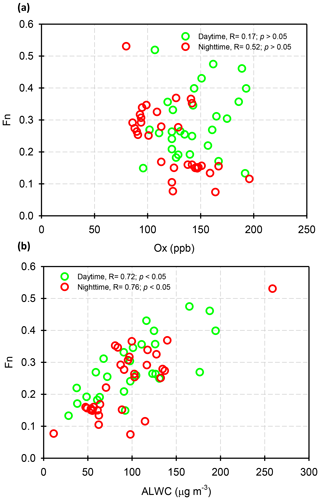Figure 7Scatter plots of (a) Fn against Ox and (b) Fn against ALWC in daytime and nighttime aerosol samples during the high hourly PM2.5 concentration conditions (hourly PM2.5≥150µg m−3).

## 3.6 Case study and production rate of ${\mathrm{NO}}_{\mathrm{3}}^{-}$ during PM2.5 episodes

Figure 8 shows several high-PM2.5 events observed from 3 to 6 March 2016. In case I, the high PM2.5 concentrations started at 18:00 LT. on 3 March and ended at 03:00 LT on 4 March. During this event, the ${\mathrm{SO}}_{\mathrm{4}}^{\mathrm{2}-}$ and ${\mathrm{NH}}_{\mathrm{4}}^{+}$ concentrations remained at almost constant levels, but the ${\mathrm{NO}}_{\mathrm{3}}^{-}$ concentrations revealed a slight enhancement. In the early morning of 4 March, the ${\mathrm{NO}}_{\mathrm{3}}^{-}$ concentrations increased from 39.4 to 47.8 µg m−3 within 4 h, resulting in a nitrate production rate of 2.3 µg m−3 h−1 (∼5.5 % h−1; the calculation of ${\mathrm{NO}}_{\mathrm{3}}^{-}$ production rate can be seen in Sect. S2). In case II, high PM2.5 concentrations were observed from 07:00 to 15:00 LT on 4 March. The ${\mathrm{NO}}_{\mathrm{3}}^{-}$ concentrations were much higher than those of ${\mathrm{SO}}_{\mathrm{4}}^{\mathrm{2}-}$, indicating nitrate-dominated aerosols. In this case, the ${\mathrm{NO}}_{\mathrm{3}}^{-}$ concentrations increased from 38.1 to 51.2 µg m−3 within 8 h, suggesting that the increasing rate of ${\mathrm{NO}}_{\mathrm{3}}^{-}$ was 1.0 µg m−3 h−1 (2.4 % h−1). Since the high ${\mathrm{NO}}_{\mathrm{3}}^{-}$ concentrations occurred under high-Ox and low-ALWC conditions, this suggested that the gas-phase reaction of NO2+OH might be the dominant source of ${\mathrm{NO}}_{\mathrm{3}}^{-}$ production in this event. In case III, a rapid growth of the PM2.5 mass was found around midnight, along with a dramatic increase in ${\mathrm{NO}}_{\mathrm{3}}^{-}$ concentrations from 23:00 LT on 4 March (31.0 µg m−3) and maximizing at 01:00 LT the next day (64.5 µg m−3). The increasing rate of ${\mathrm{NO}}_{\mathrm{3}}^{-}$ was estimated to be 11.4 µg m−3 h−1 (∼26.7 % h−1), which was much higher than those in case I and II. The high-nitrate event was found under increasing ALWC and decreasing Ox concentration conditions, suggesting that nitrate production occurred through heterogeneous processes. In Case IV, the enhancements of all SIA species coincided with increasing ALWC and declining Ox concentrations. Again, the enhancement of nitrate was likely attributed to heterogeneous reactions rather than to gas-phase processes. In this event, the ${\mathrm{NO}}_{\mathrm{3}}^{-}$ production rate was estimated to be 5.0 µg m−3 h−1 (∼15.4 % h−1).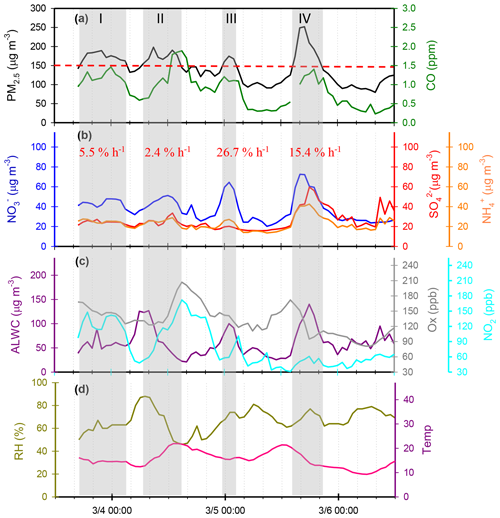Figure 8Time series of concentrations in (a) PM2.5 mass and CO, (b) SIA species (${\mathrm{NO}}_{\mathrm{3}}^{-}$, ${\mathrm{SO}}_{\mathrm{4}}^{\mathrm{2}-}$ and ${\mathrm{NH}}_{\mathrm{4}}^{+}$), (c) ALWC, Ox and NO2, and (d) RH and T in Nanjing City from 3 to 6 March 2016. The grey shadows denote PM2.5 episodes. The red numbers represent ${\mathrm{NO}}_{\mathrm{3}}^{-}$ production rate during the PM2.5 episodes.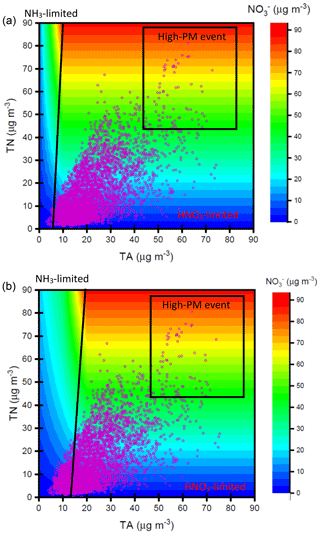Figure 9Nitrate concentrations simulated by ISORROPIA II model depending on TN and TA concentrations under (a) ${\mathrm{SO}}_{\mathrm{4}}^{\mathrm{2}-}=\mathrm{10}$µg m−3 and (b) ${\mathrm{SO}}_{\mathrm{4}}^{\mathrm{2}-}=\mathrm{60}$µg m−3. The purple dots denote the observed TN and TA concentrations at the receptor site during the sampling periods.

Through the sampling periods, a total of 12 high-PM2.5 events were found, and the ${\mathrm{NO}}_{\mathrm{3}}^{-}$ concentrations increased significantly during all the episodes (see in Table S1). Seven episodes suggested that heterogeneous processes (N2O5+H2O) might be a major pathway for nitrate formation since elevated ${\mathrm{NO}}_{\mathrm{3}}^{-}$ levels coincided with increasing ALWC and decreasing Ox (or Ox remaining at a constant level). Among these heterogeneous process events, five cases (Case III, Case IX, Case X, Case XI and Case XII in Table S1 in the Supplement) were observed during the nighttime (17:00–06:00 LT on the next day). This suggested that approximately 70 % of heterogeneous reaction of nitrate production was observed in the dark. In these events, the average ${\mathrm{NO}}_{\mathrm{3}}^{-}$ growth rate was 12.6±7.3 % h−1 (4.1±3.6µg m−3 h−1). This value was in agreement with those in the literature, in which the production rates of nitrate via heterogeneous reaction were 14.3 % h−1 by both field measurements and laboratory works (Calvert and Stockwell, 1983; Pathak et al., 2011). On the contrary, ${\mathrm{NO}}_{\mathrm{3}}^{-}$ concentrations rose with increasing Ox and decreasing ALWC in two PM2.5 episodes, indicating gas-phase processes (NO2+OH). As listed in Table S1, these gas-phase reaction cases occurred mainly during the daytime. The average production rate of ${\mathrm{NO}}_{\mathrm{3}}^{-}$ in the gas-oxidation reaction cases averaged 2.5±0.1 % h−1 (0.8±0.3µg m−3 h−1), which was in line with that (2.4 % h−1) in the subtropical polluted urban site that nitrate aerosols were mainly from gas-oxidation process (Lin and Cheng, 2007). Moreover, we also found some cases in which the elevated ${\mathrm{NO}}_{\mathrm{3}}^{-}$ might have been from both gas-phase and heterogeneous reactions, and the corresponding ${\mathrm{NO}}_{\mathrm{3}}^{-}$ growth rate was approximately 7.5±3.0 % h−1 (2.5±0.2µg m−3 h−1). In conclusion, enhancements of ${\mathrm{NO}}_{\mathrm{3}}^{-}$ in Nanjing usually occurred under increased ALWC and decreased Ox conditions, indicating that heterogeneous reactions provided the dominant pathway of nitrate formation during the PM2.5 episodes. Moreover, the average growth rate of ${\mathrm{NO}}_{\mathrm{3}}^{-}$ (12.6 % h−1) by heterogeneous processes was 5 times higher than that (2.5 % h−1) of gas-phase reactions. This might explain the abrupt increase in nitrate concentrations during the high-PM2.5 events.

## 3.7HNO3∕NH3 limitation of nitrate aerosol formation

In Nanjing, high nitrate concentrations occurred mainly under ${\mathrm{NH}}_{\mathrm{4}}^{+}$-rich regimes, indicating the involvement of atmospheric NH3. This also demonstrated that both HNO3 and NH3 were crucial precursors for particulate nitrate formation. In this section, we attempt to discuss whether HNO3 or NH3 was the limited factor for nitrate formation in Nanjing during the high-PM2.5 events. ISORROPIA II model is capable of predicting concentrations of particulate ions in addition to ALWC under thermodynamic equilibrium between gas- and aerosol-phase of these ions (Tang et al., 2016). In Sect. 3.5, we used this model to estimate ALWC. Indeed, the output data also included concentrations of ionic species. Figure S7 illustrates the scatter plots of modeled results against observations of ${\mathrm{NO}}_{\mathrm{3}}^{-}$, ${\mathrm{SO}}_{\mathrm{4}}^{\mathrm{2}-}$ and ${\mathrm{NH}}_{\mathrm{4}}^{+}$ in Nanjing during the sampling periods. Good correlations were found between modeled results and observations (R2= 0.97–0.99, with all slopes of approximately 1.0), suggesting that ISORROPIA II had a good performance in the prediction of SIA species. As a result, we can use ISORROPIA II model to test sensitivity of HNO3 and NH3 to particulate nitrate concentrations (Guo et al., 2018).

Figure 9 shows the contour plot of the simulated nitrate concentrations depending on the various total nitrate (TN) and total ammonium (TA; $\mathrm{TA}={\mathrm{NH}}_{\mathrm{3}}+{\mathrm{NH}}_{\mathrm{4}}^{+}$) levels under thermodynamic equilibrium conditions computed by ISORROPIA II model. The details of considered chemical reactions in ISORROPIA II model can be seen elsewhere (Fountoukis and Nenes, 2007). Here, sulfate concentrations were assumed to be 10 and 60 µg m−3 for the tests of different sulfate conditions. The average concentrations of total chloride ($\mathrm{HCl}+{\mathrm{Cl}}^{-}$; 1.3 µg m−3), Na+ (0.2 µg m−3), K+ (0.8 µg m−3), Mg2+ (0.1 µg m−3) and Ca2+ (0.5 µg m−3) along with ambient T (20 C) and RH (62 %) at the receptor site during the sampling period served as input data in this model. The results showed that the lower simulated ${\mathrm{NO}}_{\mathrm{3}}^{-}$ concentrations were found in the higher-${\mathrm{SO}}_{\mathrm{4}}^{\mathrm{2}-}$ case under the same TN and TA levels. This was attributed to less NH4NO3 formation under higher ${\mathrm{SO}}_{\mathrm{4}}^{\mathrm{2}-}$ conditions since ${\mathrm{SO}}_{\mathrm{4}}^{\mathrm{2}-}$ would compete with ${\mathrm{NO}}_{\mathrm{3}}^{-}$ for ${\mathrm{NH}}_{\mathrm{4}}^{+}$.

According to the simulated results, we can roughly split the plots into two parts: one is HNO3-limited area (right), and another is NH3-limited region (left) (Fig. 9). The observed TN and TA concentrations (pink circles) in Nanjing are also plotted in this figure. Most of the observed data sets were mainly affected by TN under a low-${\mathrm{SO}}_{\mathrm{4}}^{\mathrm{2}-}$ case. Under a high-${\mathrm{SO}}_{\mathrm{4}}^{\mathrm{2}-}$ condition, the observed data fell into TA-limited conditions under a low-TN and low-TA regime but fell into TN-limited conditions in high-TA and high-TN regimes. During the sampling period, high nitrate concentrations always occurred under the high-TN and high-TA conditions, highlighting that nitrate aerosol production in Nanjing during the high PM2.5 levels was mainly controlled by HNO3. Therefore, control of NOx emissions, which reduced HNO3 concentrations, might be an important way to decrease airborne nitrate concentrations and ameliorate the air quality in Nanjing.

4 Conclusion and remarks

Four intensive online measurements of water-soluble ions in PM2.5 were carried out in Nanjing City in 2016 and 2017 in order to realize the evolutions of SIA and the potential formation mechanisms of particulate nitrate. During the sampling periods, the average concentrations of ${\mathrm{NO}}_{\mathrm{3}}^{-}$, ${\mathrm{SO}}_{\mathrm{4}}^{\mathrm{2}-}$ and ${\mathrm{NH}}_{\mathrm{4}}^{+}$ were 16.7, 14.9 and 10.7 µg m−3, respectively. This indicated that ${\mathrm{NO}}_{\mathrm{3}}^{-}$ dominated the SIA. Significant seasonal variations and diurnal cycles were found for all SIA species. The low ${\mathrm{NO}}_{\mathrm{3}}^{-}$ concentrations observed during the summer daytime could be attributed to the enhanced theoretical and declined observed equilibrium constants of ${\mathrm{NO}}_{\mathrm{3}}^{-}$ and ${\mathrm{NH}}_{\mathrm{4}}^{+}$ between gas and particle phase. Obvious enhancements of ${\mathrm{NO}}_{\mathrm{3}}^{-}$ were found in terms of both absolute concentrations and relative abundances during the PM2.5 episodes, indicating that ${\mathrm{NO}}_{\mathrm{3}}^{-}$ was a major contributing species to PM2.5. Different from the results obtained in Beijing and Shanghai, high nitrate concentrations always occurred under ${\mathrm{NH}}_{\mathrm{4}}^{+}$-rich regimes. The nitrogen conversion ratio, Fn, correlated well with the ALWC but not with Ox during high-PM2.5 episodes. These findings indicated that ${\mathrm{NO}}_{\mathrm{3}}^{-}$ aerosols at the receptor site were mainly produced by heterogeneous reactions (N2O5+H2O) with the involvement of NH3 in the high-PM2.5 events. The average production rate of ${\mathrm{NO}}_{\mathrm{3}}^{-}$ from heterogeneous reactions was estimated to be 12.6 % h−1, which was 5 times higher than that of gas-phase reactions. According to the observations and ISORROPIA II simulated results, particulate nitrate formation in Nanjing was HNO3 limited, suggesting that the control of NOx emissions will be able to decrease the nitrate concentration and improve the air quality in this industrial city.

During the last decade, the mass ratios of nitrate-to-sulfate in PM2.5 in the YRD region have been found to range from 0.3 to 0.7 (Wang et al., 2003, 2006; Yang et al., 2005; Yao et al., 2002), reflecting that the ${\mathrm{SO}}_{\mathrm{4}}^{\mathrm{2}-}$ concentration was much higher than the ${\mathrm{NO}}_{\mathrm{3}}^{-}$ concentration. In the current study, the average mass ratio of nitrate-to-sulfate was 1.1. Indeed, high nitrate-to-sulfate mass ratios of >1 were also observed in other mega-cities of China recently (Ge et al., 2017; Wei et al., 2018; Ye et al., 2017; Zou et al., 2018). The elevated nitrate-to-sulfate ratio should be due to the dramatic reduction in SO2 emissions. The enhanced ratio also suggests that we should pay more attention to developing some strategies for the reduction in NOx emissions, leading to declined nitrate concentrations in the atmosphere and improvement of the air quality in China.

Data availability

All the data used in this paper are available from the corresponding author upon request (dryanlinzhang@outlook.com or zhangyanlin@nuist.edu.cn).

Supplement

Author contributions

YLZ conceived and designed the study. YCL analyzed the data and wrote the paper with YLZ. MYF and MB performed aerosol sampling and data analyses with YCL.

Competing interests

The authors declare that they have no conflict of interest.

Special issue statement

Acknowledgements

This work was supported by the Natural Scientific Foundation of China (grant nos. 41761144056, 41977305), the Provincial Natural Science Foundation of Jiangsu (No. BK20180040) and the Jiangsu Innovation & Entrepreneurship Team.

Financial support

This research has been supported by the National Key R&D Program of China (grant no. 2017YFC0212700), the Natural Scientific Foundation of China (grant nos. 41761144056, 91644103 and 41977185), and the Jiangsu Innovation & Entrepreneurship Team.

Review statement

This paper was edited by Maria Cristina Facchini and reviewed by three anonymous referees.

References

Bian, Y. X., Zhao, C. S., Ma, N., Chen, J., and Xu, W. Y.: A study of aerosol liquid water content based on hygroscopicity measurements at high relative humidity in the North China Plain, Atmos. Chem. Phys., 14, 6417–6426, https://doi.org/10.5194/acp-14-6417-2014, 2014.

Brauer, M., Hoek, G., Vliet, V. P., Meliefste, K., Fischer, P. H., Wijga, A., Koopman, L. P., Neijens, H. J., Gerritsen, J., Kerkhof, M., Heinrich, J., Bellander, T., and Brunekreef, B.: Air pollution from traffic and the development of respiratory infections and asthmatic and allergic symptoms in children, Am. J. Resp. Crit. Care, 166, 1092–1098, https://doi.org/10.1164/rccm.200108-007OC, 2002.

Brown, S. S. and Stutz, J.: Nighttime radical observation and chemistry, Chem. Soc. Rev., 41, 6405–6447, https://doi.org/10.1039/c2cs35181a, 2012.

Calvert, J. G. and Stockwell, W. R.: Acid generation in the troposphere by gas-phase chemistry, Environ. Sci. Technol., 17, 428–443, https://doi.org/10.1021/es00115a727, 1983.

Chan, C. K. and Yao, X.: Air pollution in mega cities in China, Atmos. Environ., 42, 1–42, https://doi.org/10.1016/j.atmosenv.2007.09.003, 2008.

Chang, W. L., Bhave, P. V., Brown, S. S., Riemer, N., Stutz, J., and Dabdub, D.: Heterogeneous atmospheric chemistry, ambient measurements, and model calculations of N2O5: a review, Aerosol Sci. Tech., 45, 655–685, https://doi.org/10.1080/02786826.2010.551672, 2011.

Chang, Y., Zhang, Y., Tian, C., Zhang, S., Ma, X., Cao, F., Liu, X., Zhang, W., Kuhn, T., and Lehmann, M. F.: Nitrogen isotope fractionation during gas-to-particle conversion of NOx to ${\mathrm{NO}}_{\mathrm{3}}^{\mathrm{-}}$ in the atmosphere – implications for isotope-based NOx source apportionment, Atmos. Chem. Phys., 18, 11647–11661, https://doi.org/10.5194/acp-18-11647-2018, 2018.

Defino, R. J., Siotuas, C., and Malik, S.: Potential role of ultrafine particles in associations between airborne particle mass and cardiovascular health, Environ. Health Perspect., 113, 934–938, https://doi.org/10.1289/ehp.7938, 2005.

Fountoukis, C. and Nenes, A.: ISORROPIA II: a computationally efficient thermodynamic equilibrium model for K+-Ca2+-Mg2+-${\mathrm{NH}}_{\mathrm{4}}^{+}$-Na+-${\mathrm{SO}}_{\mathrm{4}}^{\mathrm{2}-}$-${\mathrm{NO}}_{\mathrm{3}}^{\mathrm{-}}$-Cl-H2O aerosols, Atmos. Chem. Phys., 7, 4639–4659, https://doi.org/10.5194/acp-7-4639-2007, 2007.

Ge, X., Li, L., Chen, Y., Chen, H., Wu, D., Wang, J., Xie, X., Ge, S., Ye, Z., Xu, J., and Chen, M.: Aerosol characteristics and sources in Yangzhou, China resolved by offline aerosol mass spectrometry and other techniques. Environ. Pollut., 225, 74–85, https://doi.org/10.1016/j.envpol.2017.03.044, 2017.

Goodman, A. L., Underwood, G. M., and Grassian, V. H.: A laboratory study of the heterogeneous reaction of nitric acid on calcium carbonate particles. J. Geophys. Res.-Atmos., 105, 29053–29064, https://doi.org/10.1029/2000JD900396, 2000.

Griffith, S. M., Huang, X. H. H., Louie, P. K. K., and Yu, J. Z.: Characterizing the thermodynamic and chemical composition factors controlling PM2.5 nitrate: Insights from two years of online measurements in Hong Kong, Atmos. Environ., 122, 864–875, https://doi.org/10.1016/j.atmosenv.2015.02.009, 2015.

Guo, H., Xu, L., Bougiatioti, A., Cerully, K. M., Capps, S. L., Hite Jr., J. R., Carlton, A. G., Lee, S.-H., Bergin, M. H., Ng, N. L., Nenes, A., and Weber, R. J.: Fine-particle water and pH in the southeastern United States, Atmos. Chem. Phys., 15, 5211–5228, https://doi.org/10.5194/acp-15-5211-2015, 2015.

Guo, H., Otjes, R., Schlag, P., Kiendler-Scharr, A., Nenes, A., and Weber, R. J.: Effectiveness of ammonia reduction on control of fine particle nitrate, Atmos. Chem. Phys., 18, 12241–12256, https://doi.org/10.5194/acp-18-12241-2018, 2018.

He, K., Zhao, Q., Ma, Y., Duan, F., Yang, F., Shi, Z., and Chen, G.: Spatial and seasonal variability of PM2.5 acidity at two Chinese megacities: insights into the formation of secondary inorganic aerosols, Atmos. Chem. Phys., 12, 1377–1395, https://doi.org/10.5194/acp-12-1377-2012, 2012.

Huang, R.-J., Zhang, Y., Bozzetti, C., Ho, K.-F., Cao, J.-J., Han, Y., Daellenbach, R., Slowik, J. G., Platt, S. M., Canonaco, F., Zotter, P., Wolf, R., Pieber, S. M., Bruns, E. A., Crippa, M., Ciarelli, G., Piazzalunga, A., Schwikkowski, M., Abbaszade, G., Schnelle-Kreis, J., Zimmerman, R., An, Z., Szidat, S., Baltensperger, U., Haddad, I. E., and Prévôt, A. H.: High secondary aerosol contribution to particulate pollution during haze events in China, Nature, 514, 218–222, https://doi.org/10.1038/nature13774, 2014.

Huang, R. J., Chen, R., Jing, M., Yang, L., Li, Y., Chen, Q., Chen, Y., Yan, J., Lin, C., Wu, Y., Zhang, R., Haddad, J. E., Prévôt, A. S. H., O'Dowd, C. D., and Cao, J.: Source-specific health risk analysis on particulate trace elements: coal combustion and traffic emission as major contributors in wintertime Beijing, Environ. Sci. Technol., 52, 10967–10974, https://doi.org/10.1021/acs.est.8b02091, 2018.

Hui, L., Liu, X., Tan, Q., Feng, M., An. J., Qu, Y., Zhang, Y., and Jiang, M.: Characteristics, source apportionment and contribution of VOCs to ozone formation in Wuhan, Central China, Atmos. Environ., 192, 55–71, https://doi.org/10.1016/j.atmosenv.2018.08.042, 2018.

Khoder, M. I.: Atmospheric conversion of sulfur dioxide to particulate sulfate and nitrogen dioxide to particulate nitrate and gaseous nitric acid in an urban area, Chemosphere, 49, 675–684, https://doi.org/10.1016/S0045-6535(02)00391-0, 2002.

Kong, L., Yang, Y., Zhang, S., Zhao, X., Du, H., Fu, H., Zhang, S., Cheng, T., Yang, X., Chen, J., Wu, D., Sheng, J., Hong, S., and Jiao, L.: Observation of linear dependence between sulfate and nitrate in atmospheric particles, J. Geophys. Res.-Atmos., 119, 341–361, https://doi.org/10.1002/2013JD020222, 2014.

Lin, Y.-C. and Cheng, M.-T.: Evaluation of formation rates of NO2 to gaseous and particulate nitrate in the urban atmosphere, Atmos. Environ., 41, 1903–1910, https://doi.org/10.1016/j.atmosenv.2006.10.065, 2007.

Lin, Y.-C., Cheng, M.-T., Ting, W.-Y., and Yeh, C.-R.: Characteristics of gaseous HNO2, HNO3, NH3 and particulate ammonium nitrate in an urban city of central Taiwan, Atmos. Environ., 40, 4725–4733, https://doi.org/10.1016/j.atmosenv.2006.04.037, 2006.

Liu, M., Song, Y., Zhou, T., Xu, Z., Yan, C., Zheng, M., Wu, Z., Hu, M., Wu, Y., and Zhu, T.: Fine particle pH during severe haze episodes in northern China, Geophys. Res. Lett., 44, 5213–5222, https://doi.org/10.1002/2017GL073210, 2017.

Mental, T. F., Sohn, M., and Wahner, A.: Nitrate effect in the heterogeneous hydrolysis of dinitrogen pentoxide on aqueous aerosols, Phys. Chem. Chem. Phys., 1, 5451–5457, https://doi.org/10.1039/a905338g, 1999.

Nel, A.: Air pollution-related illness: effects of particles, Science, 308, 804–806, https://doi.org/10.1126/science.1108752, 2005.

Pathak, R. K., Wu, W. S., and Wang, T.: Summertime PM2.5 ionic species in four major cities of China: nitrate formation in an ammonia-deficient atmosphere, Atmos. Chem. Phys., 9, 1711–1722, https://doi.org/10.5194/acp-9-1711-2009, 2009.

Pathak, R. K., Wang, T., and Wu, W. H.: Nighttime enhancement of PM2.5 in ammonia-poor atmospheric conditions in Beijing and Shanghai: Plausible contributions of heterogeneous hydrolysis of N2O5 and HNO3 partitioning, Atmos. Environ., 45, 1183–1191, https://doi.org/10.1016/j.atmosenv.2010.09.003, 2011.

Polissar, A. V., Hopke, P. K., Paatero, P., Kaufmann, Y. J., Hall, D. K., Bodhaine, B. A., Dutton, E. G., and Harris, J. M.: The aerosol at Barrow, Alaska: Long-term trends and source locations, Atmos. Environ., 33, 2441–2458, https://doi.org/10.1016/S1352-2310(98)00423-3, 1999.

Squizzato, S., Masiol, M., Brunelli, A., Pistollato, S., Tarabotti, E., Rampazzo, G., and Pavoni, B.: Factors determining the formation of secondary inorganic aerosol: a case study in the Po Valley (Italy), Atmos. Chem. Phys., 13, 1927–1939, https://doi.org/10.5194/acp-13-1927-2013, 2013.

Tang, X., Zhang, X., Ci, Z., Guo, J., and Wang, J.: Speciation of the major inorganic salts in atmospheric aerosols of Beijing: China: measurements and comparison with model, Atmos. Environ., 133, 123–134, https://doi.org/10.1016/j.atmosenv.2016.03.013, 2016.

Tao, Y., Ye, X., Ma, Z., Xie, Y., Wang, R., Chen, J., Yang, X., and Jiang, S.: Insights into different nitrate formation mechanisms from seasonal variations of secondary inorganic aerosols in Shanghai, Atmos. Environ., 145, 1–9, https://doi.org/10.1016/j.atmosenv.2016.09.012, 2016.

ten Brink, H., Otjes, R., Jongejan, P., and Slanina, S.: An instrument for semi-continuous monitoring of the size-distribution of nitrate, ammonium, sulfate and chloride in aerosols, Atmos. Environ., 41, 2768–2779, https://doi.org/10.1016/j.atmosenv.2006.11.041, 2007.

Wahner, A., Mental, T. F., Sohn, M., and Stier, J.: Heterogenous reaction of N2O5 on sodium nitrate aerosol, J. Geophys. Res.-Atmos., 103, 31103–31112, https://doi.org/10.1029/1998JD100022, 1998.

Wang, G., Wang, H., Yu, Y., Gao, S., Feng, J., Gao, S., and Wang, L.: Chemical characterization of water-soluble components of PM10 and PM2.5 atmospheric aerosols in five locations of Nanjing, China, Atmos. Environ., 37, 2893–2902, https://doi.org/10.1016/S1352-2310(03)00271-1, 2003.

Wang, G., Zhang, R., Geomez, M. E., Yang, L., Zamora, M. L., Hu, M., Lin, Y., Peng, J., Guo, S., Meng, J., Li, J., Cheng, C., Hu, T., Ren, Y., Wang, Y., Gao, J., An, Z., Zhou, W., Li, G., Wang, J., Tian, P., Marrero-Ortiz, W., Secrest, J., Du, Z., Zheng, J., Shang, D., Zheng, L., Shao, M., Wang, W., Huang, Y., Wang, Y., Zhu, Y., Li, Y., Hu, J., Pan, B., Cai, L., Cheng, Y., Ji, Y., Zhang, F., Rosenfeld, D., Liss, P. S., Duce, R. A., Kolb, C. E., and Molina, M. J.: Persistent sulfate formation from London fog to Chinese haze, P. Natl. Acad. Sci. USA, 113, 13630–13635, https://doi.org/10.1073/pnas.1616540113, 2016.

Wang, H., Zhu, B., Shen, L., Xu, H., An, J., Xue, G., and Cao, J.: Water soluble ions in atmospheric aerosols measured in five sites in the Yantze River Delta, China: size-fractionated seasonal variation and sources, Atmos. Environ., 123, 370–379, https://doi.org/10.1016/j.atmosenv.2015.05.070, 2015.

Wang, H., Lu, K., Chen, X., Zhu, Q., Chen, Q., Guo, S., Jiang, M., Li, X., Shang, D., Tang, Z., Wu, Y., Wu, Z., Zou, Q., Zheng, Y., Zheng, L., Zhu, T., Hu, M., and Zhang, Y.: High N2O5 concentrations observed in urban Beijing: implications of a large nitrate formation, Environ. Sci. Tech. Let., 4, 416–420, https://doi.org/10.1021/acs.estlett.7b00341, 2017.

Wang, W., Yu, J., Cui. Y., He, J., Xue, P., Cao, W., Ying, H., Gao, W., YIng, Y., Gao, W., Yan, Y., Hu, B., Xin, J., Wang, L., Liu, Z., Sun, Y., Ji, D., and Wang, Y.: Characteristics of fine particulate matter and its sources in an industrialized coastal city, Ningbo, Yantze River Delta, China, Atmos. Res., 203, 105–117, https://doi.org/10.1016/j.atmosres.2017.11.033, 2018.

Wang, Y., Zhuang, G., Zhang, X., Xu, C., Tang, A., Chen, J., and An, Z.: The ion chemistry, seasonal cycle, and sources of PM2.5 and TSP aerosol in Shanghai, Atmos. Environ., 40, 2935–2952, https://doi.org/10.1016/j.atmosenv.2005.12.051, 2006.

Wei, L., Yue, S., Zhao, W., Yang, W., Zhang, Y., Ren, L., Han, X., Guo, Q., Sun, Y., Wang, Z., and Fu, P.: Stable sulfur isotope ratios and chemical compositions of fine aerosols (PM2.5) in Beijing, China, Sci. Total Environ., 633, 1156–1164, https://doi.org/10.1016/j.scitotenv.2018.03.153, 2018.

Wen, L., Chen, J., Yang, L., Wang, X., Xu, C., Sui, X., Yao, L., Zhu, Y., Zhang, J., Zhu, T., and Wang, W.: Enhanced formation of particulate nitrate at a rural site on the North China Plain in summer: the importance roles of ammonia and ozone, Atmos. Environ., 101, 294–302, https://doi.org/10.1016/j.atmosenv.2014.11.037, 2015.

Yang, H., Yu, J. Z., Ho, S. S. H., Xu, J., Wu, W.-S., Wan, C. H., Wang, X., Wang, X., and Wang, L.: The chemical composition of inorganic and carbonaceous materials in PM2.5 in Nanjing, China, Atmos. Environ., 39, 3735–3749, https://doi.org/10.1016/j.atmosenv.2005.03.010, 2005.

Yao, X., Chan, C. K., Fang, M., Cadle, S., Chan, T., Mulawa, P., He, K., and Ye, B.: The water-soluble ionic composition PM2.5 in Shanghai and Beijing, China, Atmos. Environ., 36, 4223–4234, https://doi.org/10.1016/j.atmosenv.2005.12.051, 2002.

Ye, X. N., Ma, Z., Zhang, J. C., Du, H. H., Chen, J. M., Chen, H., Yang, X., Gao, W., and Geng, F. H.: Important role of ammonia on haze formation in Shanghai, Environ. Res. Lett., 6, 024019, https://doi.org/10.1088/1748-9326/6/2/024019, 2011.

Ye, Z., Liu, J., Gu, A., Feng, F., Liu, Y., Bi, C., Xu, J., Li, L., Chen, H., Chen, Y., Dai, L., Zhou, Q., and Ge, X.: Chemical characterization of fine particulate matter in Changzhou, China, and source apportionment with offline aerosol mass spectrometry, Atmos. Chem. Phys., 17, 2573–2592, https://doi.org/10.5194/acp-17-2573-2017, 2017.

Zhang, R., Jing, J., Tao, J., Hsu, S.-C., Wang, G., Cao, J., Lee, C. S. L., Zhu, L., Chen, Z., Zhao, Y., and Shen, Z.: Chemical characterization and source apportionment of PM2.5 in Beijing: seasonal perspective, Atmos. Chem. Phys., 13, 7053–7074, https://doi.org/10.5194/acp-13-7053-2013, 2013.

Zhang, Y.-L. and Cao, F.: Fine particulate matters (PM2.5) in China at a city level, Sci. Rep.-UK, 5, 14884, https://doi.org/10.1038/srep14884, 2015.

Zhao, P. S., Dong, F., He, D., Zhao, X. J., Zhang, X. L., Zhang, W. Z., Yao, Q., and Liu, H. Y.: Characteristics of concentrations and chemical compositions for PM2.5 in the region of Beijing, Tianjin, and Hebei, China, Atmos. Chem. Phys., 13, 4631–4644, https://doi.org/10.5194/acp-13-4631-2013, 2013.

Zheng, B., Tong, D., Li, M., Liu, F., Hong, C., Geng, G., Li, H., Li, X., Peng, L., Qi, J., Yan, L., Zhang, Y., Zhao, H., Zheng, Y., He, K., and Zhang, Q.: Trends in China's anthropogenic emissions since 2010 as the consequence of clean air actions, Atmos. Chem. Phys., 18, 14095–14111, https://doi.org/10.5194/acp-18-14095-2018, 2018.

Zou, J., Liu, Z., Hu, B., Huang, X., Wen, T., Ji, D., Liu, J., Yang, Y., Yao, Q., and Wang, Y.: Aerosol chemical compositions in the Northern China Plain and the impact on visibility in Beijing and Tianjin, Atmos. Res., 201, 235–246, https://doi.org/10.1016/j.atmosres.2017.09.014, 2018.